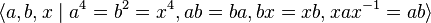# SmallGroup(32,2)

View a complete list of particular groups (this is a very huge list!)[SHOW MORE]

## Definition

This group is a semidirect product$(Z_4 \times Z_2) \rtimes Z_4$, and can be defined by the following presentation:$\langle a, b, x \mid a^4 = b^2 = x^4, ab = ba, bx = xb, xax^{-1} = ab\rangle$

See the section #Description by presentation for how to construct the group using this presentation in GAP.

## Arithmetic functions

Want to compare and contrast arithmetic function values with other groups of the same order? Check out groups of order 32#Arithmetic functions
Function Value Similar groups Explanation for function value GAP verification (set G := SmallGroup(32,2);) -- see more at #GAP implementation
underlying prime of p-group 2
order (number of elements, equivalently, cardinality or size of underlying set) 32 groups with same order Order(G); using Order.
prime-base logarithm of order 5 groups with same prime-base logarithm of order
max-length of a group 5 max-length of a group equals prime-base logarithm of order for group of prime power order
chief length 5 chief length equals prime-base logarithm of order for group of prime power order
composition length 5 composition length equals prime-base logarithm of order for group of prime power order
exponent of a group 4 groups with same order and exponent of a group | groups with same prime-base logarithm of order and exponent of a group | groups with same exponent of a group Exponent(G); using Exponent.
prime-base logarithm of exponent 2 groups with same order and prime-base logarithm of exponent | groups with same prime-base logarithm of order and prime-base logarithm of exponent | groups with same prime-base logarithm of exponent
Frattini length 2 groups with same order and Frattini length | groups with same prime-base logarithm of order and Frattini length | groups with same Frattini length FrattiniLength(G); using FrattiniLength.
nilpotency class 2 groups with same order and nilpotency class | groups with same prime-base logarithm of order and nilpotency class | groups with same nilpotency class NilpotencyClassOfGroup(G); using NilpotencyClassOfGroup.
derived length 2 groups with same order and derived length | groups with same prime-base logarithm of order and derived length | groups with same derived length DerivedLength(G); using DerivedLength.
minimum size of generating set 2 groups with same order and minimum size of generating set | groups with same prime-base logarithm of order and minimum size of generating set | groups with same minimum size of generating set Rank(G); using Rank.
subgroup rank of a group 3 groups with same order and subgroup rank of a group | groups with same prime-base logarithm of order and subgroup rank of a group | groups with same subgroup rank of a group
rank of a p-group 3 groups with same order and rank of a p-group | groups with same prime-base logarithm of order and rank of a p-group | groups with same rank of a p-group RankAsPGroup(G); using RankAsPGroup.
normal rank of a p-group 3 groups with same order and normal rank of a p-group | groups with same prime-base logarithm of order and normal rank of a p-group | groups with same normal rank of a p-group NormalRank(G); using NormalRank.
characteristic rank of a p-group 3 groups with same order and characteristic rank of a p-group | groups with same prime-base logarithm of order and characteristic rank of a p-group | groups with same characteristic rank of a p-group CharacteristicRank(G); using CharacteristicRank.

## Group properties

Want to compare and contrast group properties with other groups of the same order? Check out groups of order 32#Group properties
Property Satisfied? Explanation Comment
group of prime power order Yes
nilpotent group Yes prime power order implies nilpotent
supersolvable group Yes via nilpotent: finite nilpotent implies supersolvable
solvable group Yes via nilpotent: nilpotent implies solvable
abelian group No
T-group No
monolithic group No
group of nilpotency class two Yes
metabelian group Yes
finite group that is 1-isomorphic to an abelian group Yes via cocycle skew reversal generalization of Baer correspondence

## GAP implementation

### Group ID

This finite group has order 32 and has ID 2 among the groups of order 32 in GAP's SmallGroup library. For context, there are 51 groups of order 32. It can thus be defined using GAP's SmallGroup function as:

SmallGroup(32,2)

For instance, we can use the following assignment in GAP to create the group and name it$G$:

gap> G := SmallGroup(32,2);

Conversely, to check whether a given group$G$ is in fact the group we want, we can use GAP's IdGroup function:

IdGroup(G) = [32,2]

or just do:

IdGroup(G)

to have GAP output the group ID, that we can then compare to what we want.

### Description by presentation

gap> F := FreeGroup(3);
<free group on the generators [ f1, f2, f3 ]>
gap> G := F/[F.1^4,F.2^4,F.3^2,Comm(F.1,F.2)*F.3^(-1),Comm(F.1,F.3),Comm(F.2,F.3)];
<fp group on the generators [ f1, f2, f3 ]>
gap> IdGroup(G);
[ 32, 2 ]

### GAP verification of function values and group properties

Below is a GAP implementation verifying the various function values and group properties as stated in this page. Before beginning, set G := SmallGroup(32,2); or set it to this group in any other manner:

gap> Order(G);
32
gap> Exponent(G);
4
gap> NilpotencyClassOfGroup(G);
2
gap> DerivedLength(G);
2
gap> FrattiniLength(G);
2
gap> Rank(G);
2
gap> RankAsPGroup(G);
3
gap> NormalRank(G);
3
gap> CharacteristicRank(G);
3